## Deep Learning with Keras and TensorFlow

Certification Training
3853 Learners
9 Chapters +

# Convolutional Neural Networks Tutorial

Welcome to the seventh lesson, ‘Convolutional Neural Networks’ of the Deep Learning Tutorial, which is a part of the Deep Learning (with TensorFlow) Certification Course offered by Simplilearn.

This lesson focuses on Convolutional Neural Networks along with its process, working for image recognition, pooling concepts, and implementation.

Let us begin with the objectives of this lesson.

## Objectives

After completing this lesson on Convolutional Neural Networks, you’ll be able to:

• Learn how to implement CNNs within TensorFlow

• Discuss the process of convolution and how it works for image processing or other tasks

• Describe what CNNs are and their applications

• Illustrate how zero padding works with variations in kernel weights

• Elaborate the pooling concepts in CNNs

• Explain how to calculate the weighted inputs for all the feature maps stacked together

• Explain how CNNs differ from ANNs

In the next section, let us talk about CNN and their uses.

## Convolutional Neural Network (CNN) and Their Uses

Convolutional Neural Networks (CNN) are neural networks mainly used for image processing and classification.

Until quite recently, computers were not good at tasks like recognizing puppy in a picture or recognizing spoken words, which humans excel at.

The human brain uses special visual, auditory or other sensory modules to process tasks even before the sensory information reaches consciousness.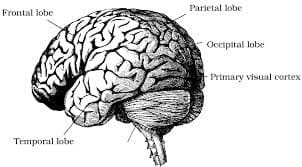CNNs are based on the brain’s visual cortex function.

Convolutional Neural Networks are also popularly known as ConvNets/CovNets.

In the next section, let us discuss the applications of CNN.

Preparing for a career in Deep Learning? Check out our Course Preview now!

## Applications of Convolutional Neural Network (CNN)

The applications of CNN are listed as follows:

• Image search

• Self-driving cars

• Automatic video classification systems

• Voice recognition

• Natural language processing

There has been a lot of progress with CNNs in various image tasks like:

• Object detection

• Detecting if an image has a person, animal or vehicle etc

• Object localization

• Zeroing in on the object of interest in the image - For example, detecting the person in the image and drawing a boundary around it

• Image segmentation

• Here the network outputs an image where each pixel indicates the class of the object to which the corresponding input pixel belongs

NOTE: In Object localization, the network outputs bounding boxes around various objects. This typically needs a CNN followed by an RNN scheme.

In the next section, let us understand the difference between ANN and CNN.

## Difference between ANN and CNN

In an ANN, each neuron in the network is connected to every other neuron in the adjacent hidden layers.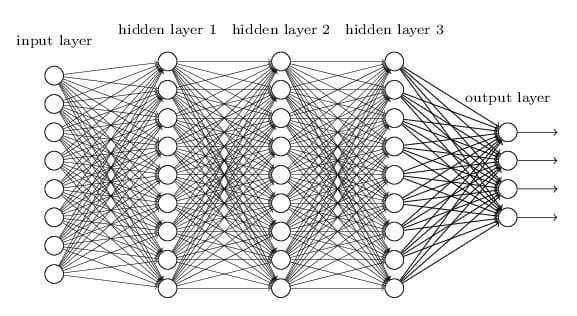In a CNN, each neuron in the hidden layer is connected to a small region of the input neurons.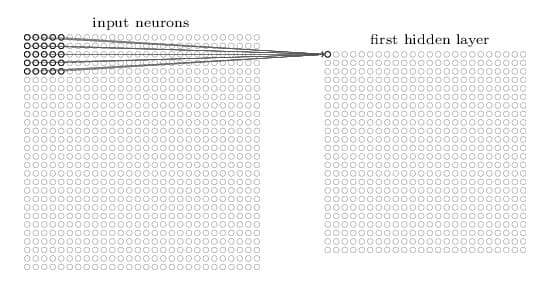Why not use regular ANNs for image tasks?

For small images, it might work, but for large images the no of pixels are so many leading to millions of connections between neurons, leading to intractable solutions.

Let us study CNN Architecture in the next section.

## Convolutional Neural Network (CNN) Architecture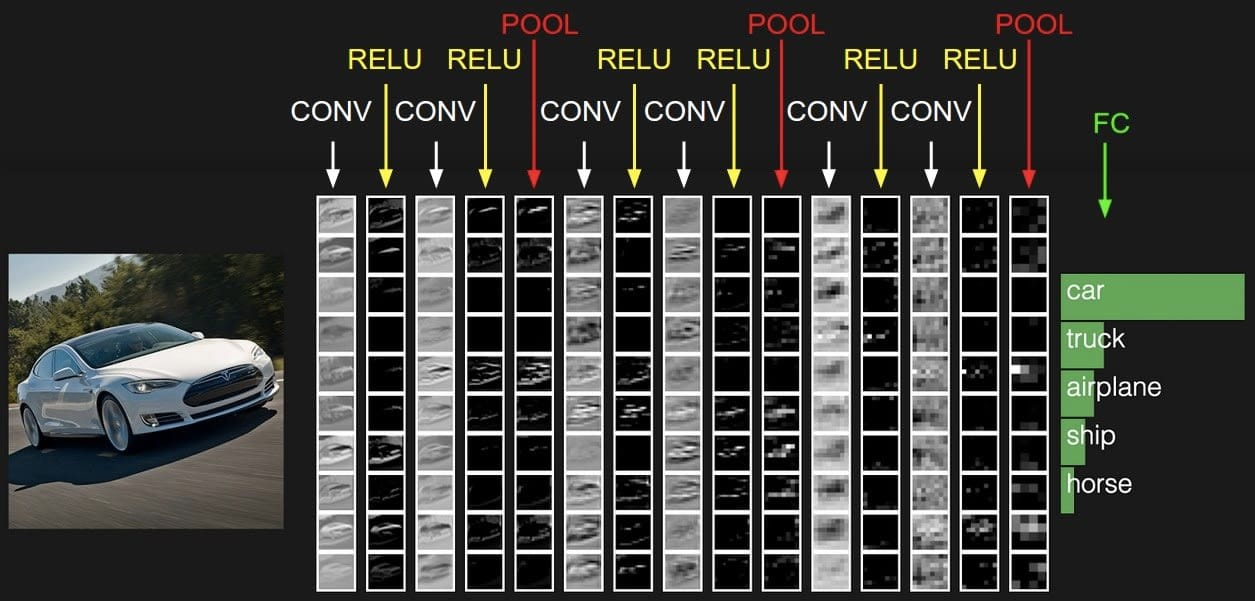CNN architecture has the following configuration:

• Convolutional Layer

• Pooling Layer

• Fully connected Layer

Most normal configuration is the convolutional layer, followed by ReLU layer, followed by a pooling layer. The sets then keep repeating.

The final layer is the fully connected layer, which precedes the final image classification.

In the next section, let us focus on the other architectures of CNN.

## Other Architectures of Convolutional Neural Network (CNN)

Over the years, many variants of this basic architecture have been developed such as:

• LeNet-5 (1998)

• AlexNet (2012)

• ZFNet (2013)

• VGGNet(2014)

• ResNet (2015)

These models can be used in applications by developers.

Let us discuss the Convolution process in the next section.

## Convolution Process

The Convolution Process has been discussed in the following sections.

### Convolutional Layer

Here neurons in the first hidden/convolutional layer are connected only to a subset of neurons in the prior layer, and so on in the next convolutional layer. This area in the input image is called Receptive Field.

CNN layers with rectangular local receptive fields are shown below: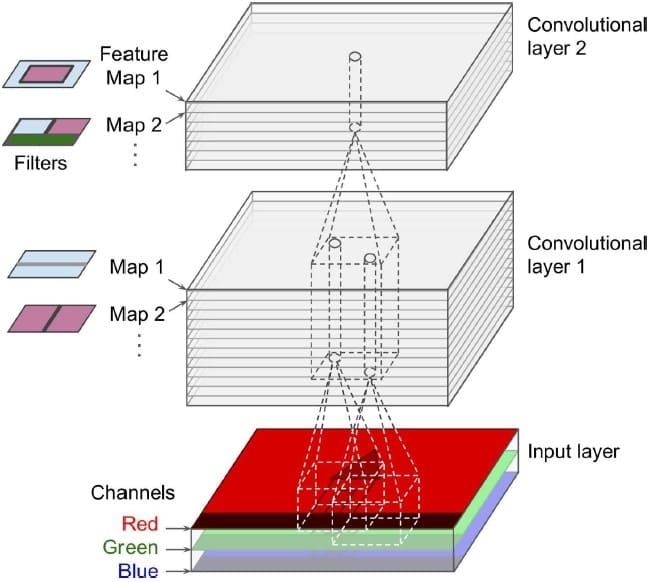### High And Low-level Features

First, the hidden layer focuses on lower-level features and the later layers assemble them into higher-level features.

Consider the image of a dog whose features can be categorized as:

• High-level features - Eyes/ears/mouth/paws

• Low-level features - Edges/curves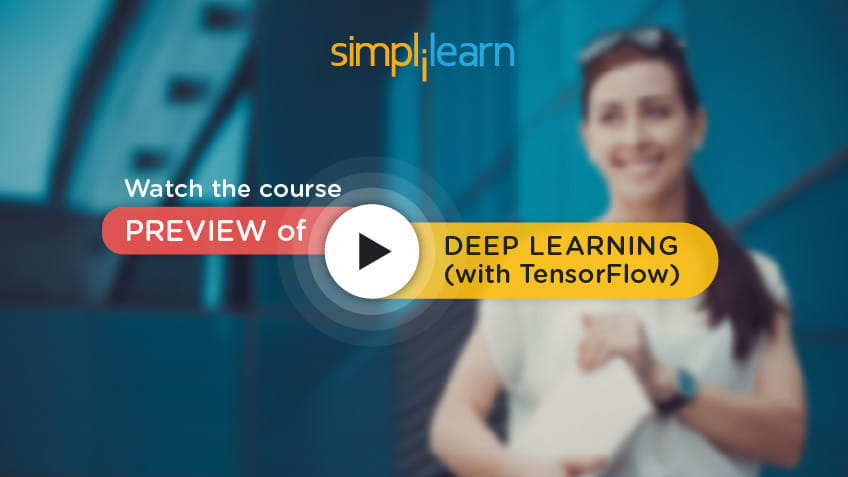## Process of Convolution

Imagine a small patch being slid across the input image. This sliding is called convolving.

It is similar to a flashlight moving from the top left end progressively scanning the entire image. This patch is called the filter/kernel. The area under the filter is the receptive field.

The idea is to detect local features in a smaller section of the input space, section by section to eventually cover the entire image.

In other words, the CNN layer neurons depend only on nearby neurons from the previous layer. This has the impact of discovering the features in a certain limited area of the input feature map.

Example

Assume the filter/kernel is a weight matrix “wk“. For example, let’s assume a 3X3 weighted matrix.

 0 1 1 1 0 0 1 0 1

The weight matrix is a filter to extract some particular features from the original image. It could be for

extracting curves, identifying a specific color, or recognizing a particular voice.

Assume the input to be a 6X6 image.

 81 2 209 44 71 58 24 56 108 98 12 112 91 0 189 65 79 232 12 0 0 5 1 71 2 32 23 58 8 209 49 98 81 112 54 9

As the filter/kernel is slid across the input layer, the convolved layer is obtained by adding the values

obtained by element-wise multiplication of the weight matrix.

Input layer

 81 2 209 44 71 58 24 56 108 98 12 112 91 0 189 65 79 232 12 0 0 5 1 71 2 32 23 58 8 209 49 98 81 112 54 9

Filter/Kernel (Weighted matrix)

 0 1 1 1 0 0 1 0 1

Output

 515

For example, when the weighted matrix starts from the top left corner of the input layer, the output value is calculated as:

(81x0+2x1+209x1)+(24x1+56x0+108X0)+(91x1+0x0+189x1) = 515

The filter then moves by 1 pixel to the next receptive field and the process is repeated. The output

layer obtained after the filter slides over the entire image would be a 4X4 matrix.

This is called an activation map/ feature map.

Input layer

 81 2 209 44 71 58 24 56 108 98 12 112 91 0 189 65 79 232 12 0 0 5 1 71 2 32 23 58 8 209 49 98 81 112 54 9

Filter/Kernel (Weighted matrix)

 0 1 1 1 0 0 1 0 1

Output (Activation/Feature Map)

 515 374

The distance between two consecutive receptive fields is called the stride.

In this example, the stride is 1 since the receptive field was moved by 1 pixel at a time.

Spacing of Strides

It is also possible to connect a large input layer to a much smaller layer by spacing out the receptive fields, i.e., increasing the stride.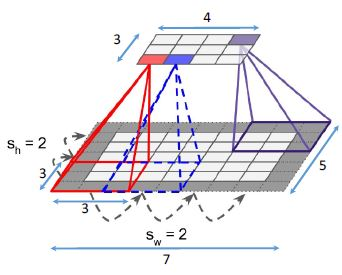In the diagram :

• The Input layer is 5x7

• The Output layer is 3x4

• The Receptive field is 3x3

• The Stride is 2

Here stride is same across two dimensions, but in general, it can be different across height “sh” and width “sw”.

The following image depicts Reducing dimensionality using a stride:

Vertical And Horizontal Filters

The image shows two kernels – vertical and horizontal filters. Each is a 5x5 matrix with all 0s, except 1 in the vertical line for vertical filter and 1 in a horizontal line in a horizontal filter.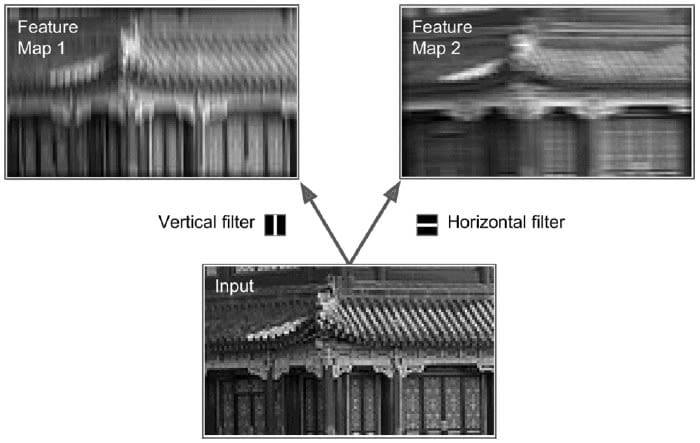Vertical Filter:

 0 0 1 0 0 0 0 1 0 0 0 0 1 0 0 0 0 1 0 0 0 0 1 0 0

Horizontal Filter:

 0 0 0 0 0 0 0 0 0 0 1 1 1 1 1 0 0 0 0 0 0 0 0 0 0

The effect of multiplying with vertical kernel filter is that all pixels except the vertical lines get

subdued. Similarly, with horizontal kernel filter, it accentuates the horizontal lines.

The output image has a feature map, which highlights the areas in the image that are most similar to the filter.

In this fashion, a CNN finds low-level features first and then combines them in higher layers to

detect complex features at points where both filters are active.

In the next section, let us focus on Zero Padding.

What are you waiting for? Interested in taking up a Deep Learning Course? Check out our Course Preview here!

A neuron located in row i, column j of a given layer is connected to neurons in the previous layer located in rows i to i+fh-1, columns j to j+fw-1, where fh and fw are the height and width of the receptive field.

To maintain the height and width dimensions of the convolutional layer the same as the previous layer, one zero-pads the input layer.

The following image shows the CNN layer with zero padding: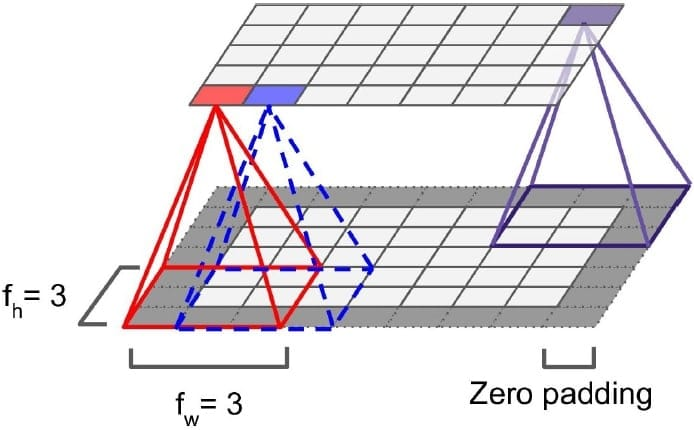CALCULATION

1. Assume the input image to be of size 32x32x3

2. The filter size is 5x5x3

3. The required output image is 32x32x3

4. Zero Padding = (K − 1)/2

where K= filter size

1. Zero Padding = (5 − 1)/2

= 2

Therefore the input is zero padded with the value of 2 resulting in a matrix of 36x36x3

OUTPUT SIZE

The output size can be calculated as:

### Find our Deep Learning with Keras and TensorFlow Online Classroom training classes in top cities:

Name Date Place
Deep Learning with Keras and TensorFlow 10 Apr -2 May 2020, Weekdays batch Your City View Details
Deep Learning with Keras and TensorFlow 25 Apr -17 May 2020, Weekend batch San Francisco View Details
Deep Learning with Keras and TensorFlow 16 May -7 Jun 2020, Weekend batch Dallas View Details
Related Courses
Learner Reviews
Related Articles
• Disclaimer
• PMP, PMI, PMBOK, CAPM, PgMP, PfMP, ACP, PBA, RMP, SP, and OPM3 are registered marks of the Project Management Institute, Inc.

Name*
Email*
Phone Number*